You can download the lectures here. We will try to upload lectures prior to their corresponding classes.

•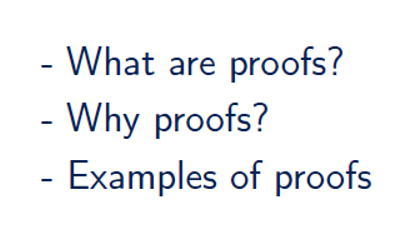An introduction to 301
tl;dr: We discuss the structure of the course, additional resources, grading policy, responsibilities, support in the first half. In the second half, we discuss what proofs are and why we need them.
[slides]

Suggested Readings: Chapter 0 of the textbook

•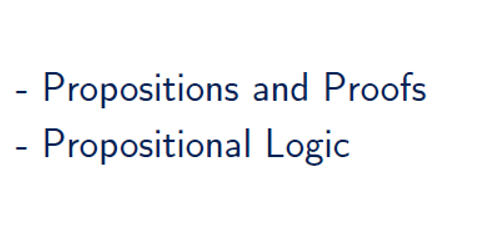Propositional logic
tl;dr: In this lecture, we shall introduce propositional logic and we shall use it to analyze the forms of proofs.
[slides]

Suggested Readings: Section 1.1. of the textbook

Extra exercises: PDF

•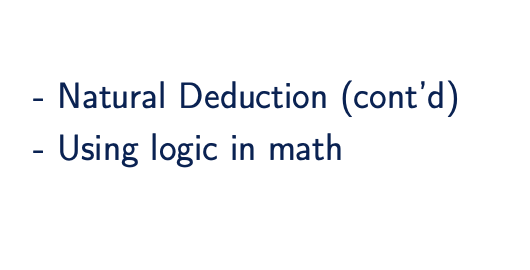Natural Deduction, Excluded Middle, and Truth Tables
tl;dr: We shall introduce the inference rules of disjunction, contradiction and negation. We shall also talk about the semantics of (classical) propositional logic.
[slides] [handout]

Suggested Readings: Section 1.1. of the textbook

Appendix D of the textbook

Extra exercises: PDF

•Propositions with Lean
tl;dr: We use the proof assistant Lean as propositional logic.
[Lean code]

Open the attached lean code file with your favourite text editor. I recommend Sublime text or VSCode.

Extras

•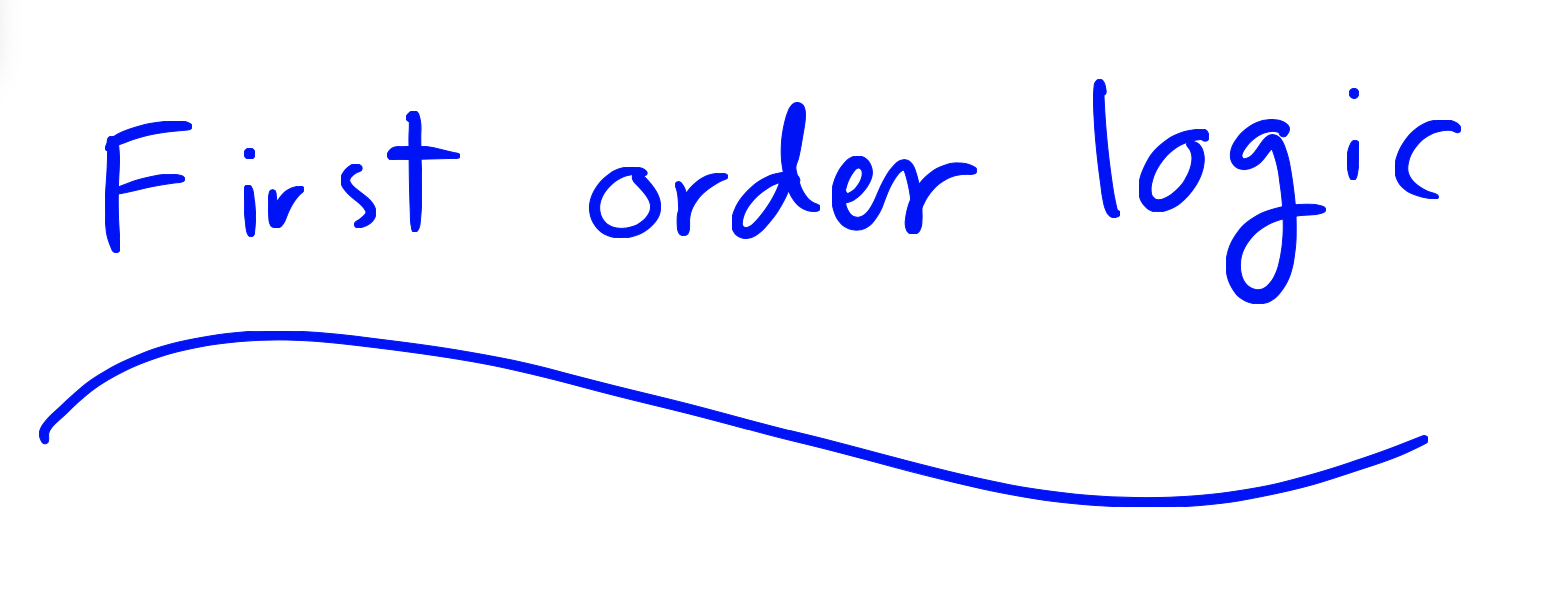First Order Logic -- Variables, Predicates, and Quantifiers
tl;dr: We explore some of the limitations of the propositional logic. We see how the first order logic gives us the right tools to overcome these limitations.
[slides]

Suggested Readings: Sections 1.2 and 1.3 of the textbook

•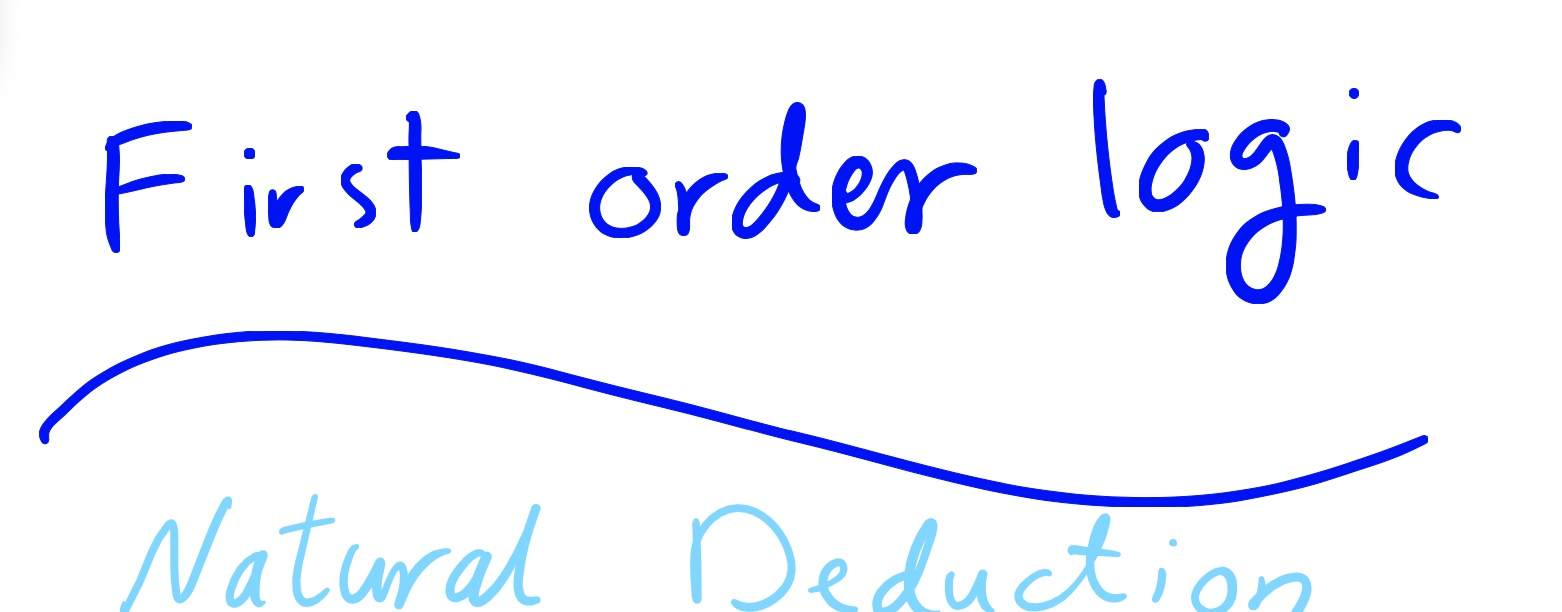First Order Logic -- Natural Deduction
tl;dr: We introduce natural deduction for first order logic.
[slides]

Suggested Readings: Sections 1.2 and 1.3 of the textbook

•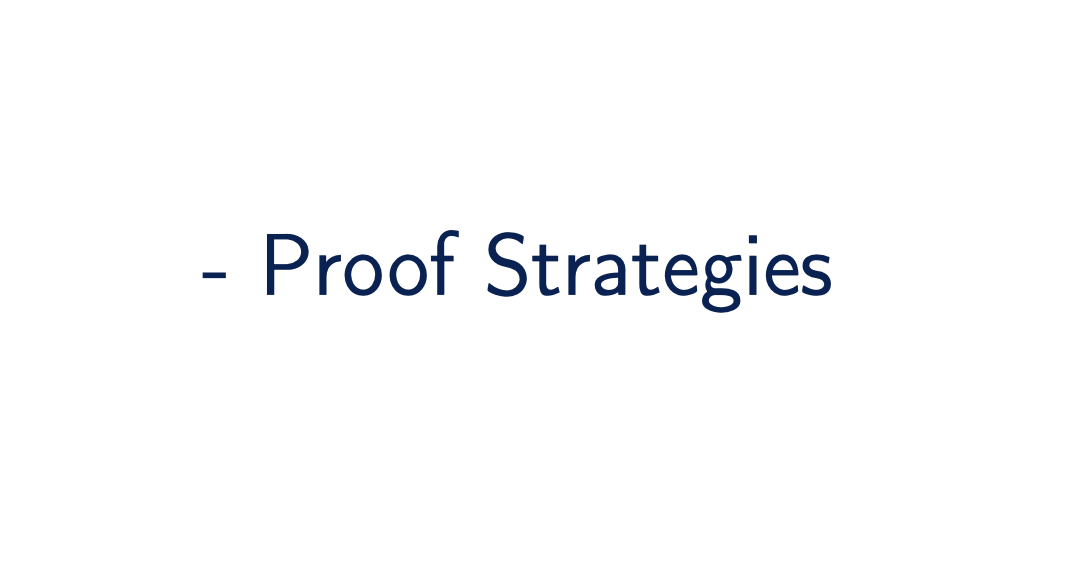Proof Strategies
tl;dr: We use natural deduction to get a bird's eye view of the structure of our mathematical proofs.
[slides]
•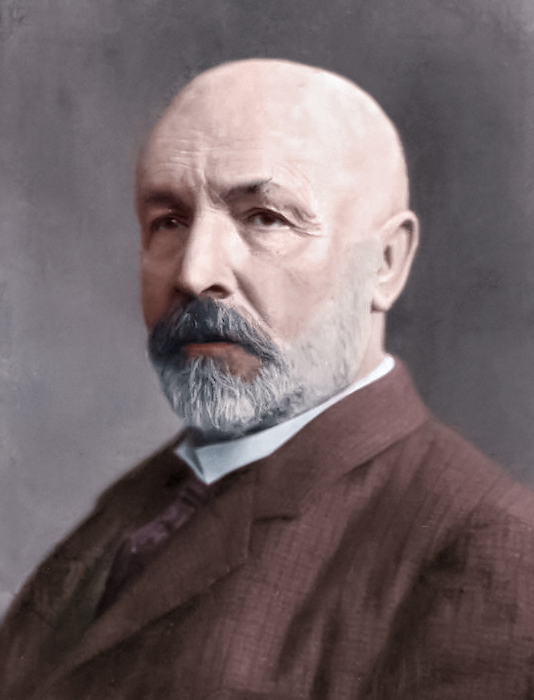Sets (part I)
tl;dr: We make the idea of collections and containment precise by introducing the notion of set.
[slides] [handout]

Suggested Readings: Chapter 2 of the textbook

•Sets (part II)
tl;dr: We make the idea of collections and containment precise by introducing the notion of set.
[slides] [handout]

Suggested Readings: Chapter 2 of the textbook

•Sets (part III)
tl;dr: We make the idea of collections and containment precise by introducing the notion of set.
[slides] [handout]
• Chapter 2 of the textbook
•Sets (part IV)
tl;dr: We make the idea of collections and containment precise by introducing the notion of set.
[slides] [handout]
• Chapter 2 of the textbook
•Relations
tl;dr: We introduce the notions of ordered sets, partially ordered sets, and equivalence relations with tons of examples.
[slides]

Suggested Readings: Chapters 3 and 5 of the textbook

•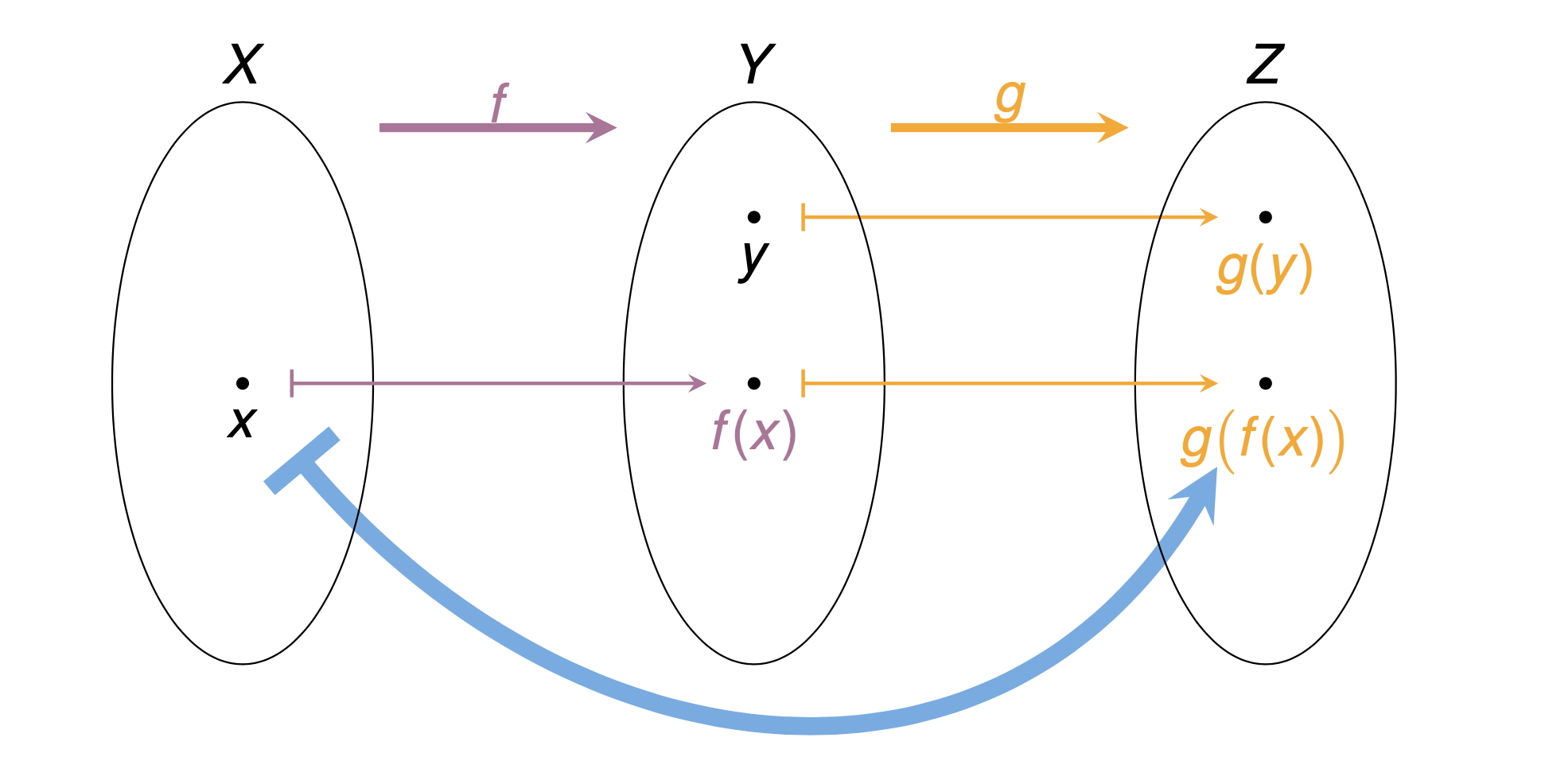Functions
[slides] [handout]

Suggested Readings: Chapters 3 and 5 of the textbook

•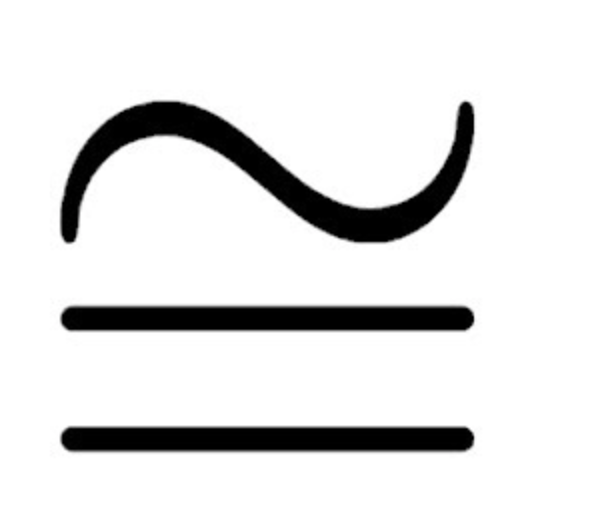Isomorphisms
tl;dr: When are two sets the same? Take 2
[slides] [handout]

Suggested Readings: Chapters 3 and 5 of the textbook

Extras

•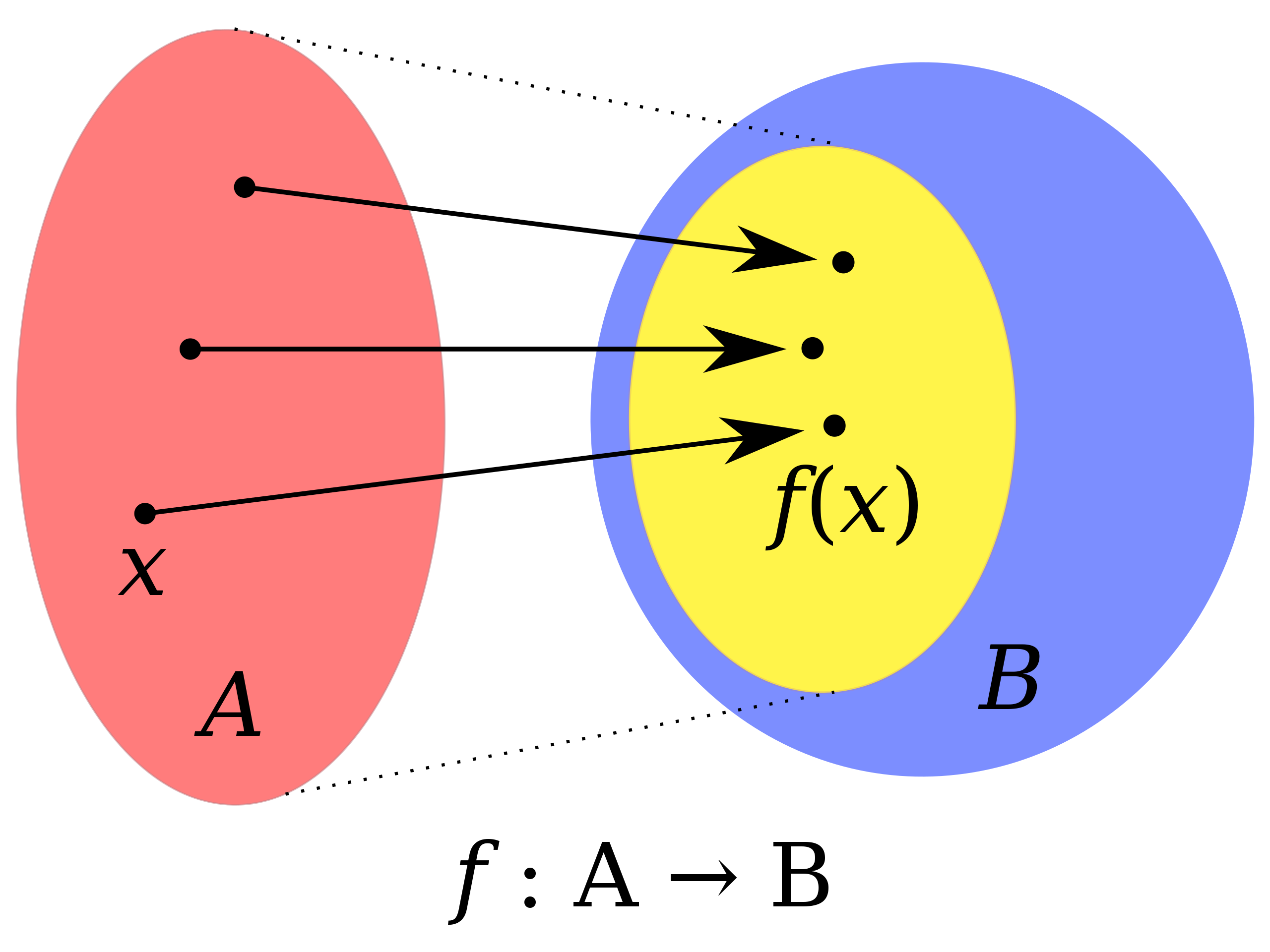Images and pre-images
tl;dr: We learn about images, pre-images, and fibres of functions. We also learn about image factorization. In the second part, we encounter axiom of choice.
[slides] [handout]

Suggested Readings: Chapters 3 and 5 of the textbook

Extras

More to come …

•Induction on natural numbers
tl;dr: We learn about natural numbers and the principles of induction and least element.
[notes]

Suggested Readings: Chapter 4 of the textbook

•Recursion
tl;dr: We learn about recursion. We use recursion to define addition(+) and we prove 1+1=2.
[slides] [handout]

Suggested Readings: Chapter 4 of the textbook

Cool stuff to do with recursion: Recursion ‘Super Power’ (in Python) - Computerphile

• Integers
tl;dr: We construct integers from natural numbers and define arithmetic operations on them.
[slides] [handout]

Suggested Readings: Section B.2 of the textbook

• Rational numebrs
tl;dr: We construct rational numebrs from integers in two ways and prove they are the same.
[slides] [handout]